# 978-0134292380 Chapter 4 Part 1

Document Type
Test Prep
Book Title
Fundamentals of Hydraulic Engineering Systems 5th Edition
Authors
A. Osman H. Akan, Ned H. C. Hwang, Robert J. Houghtalen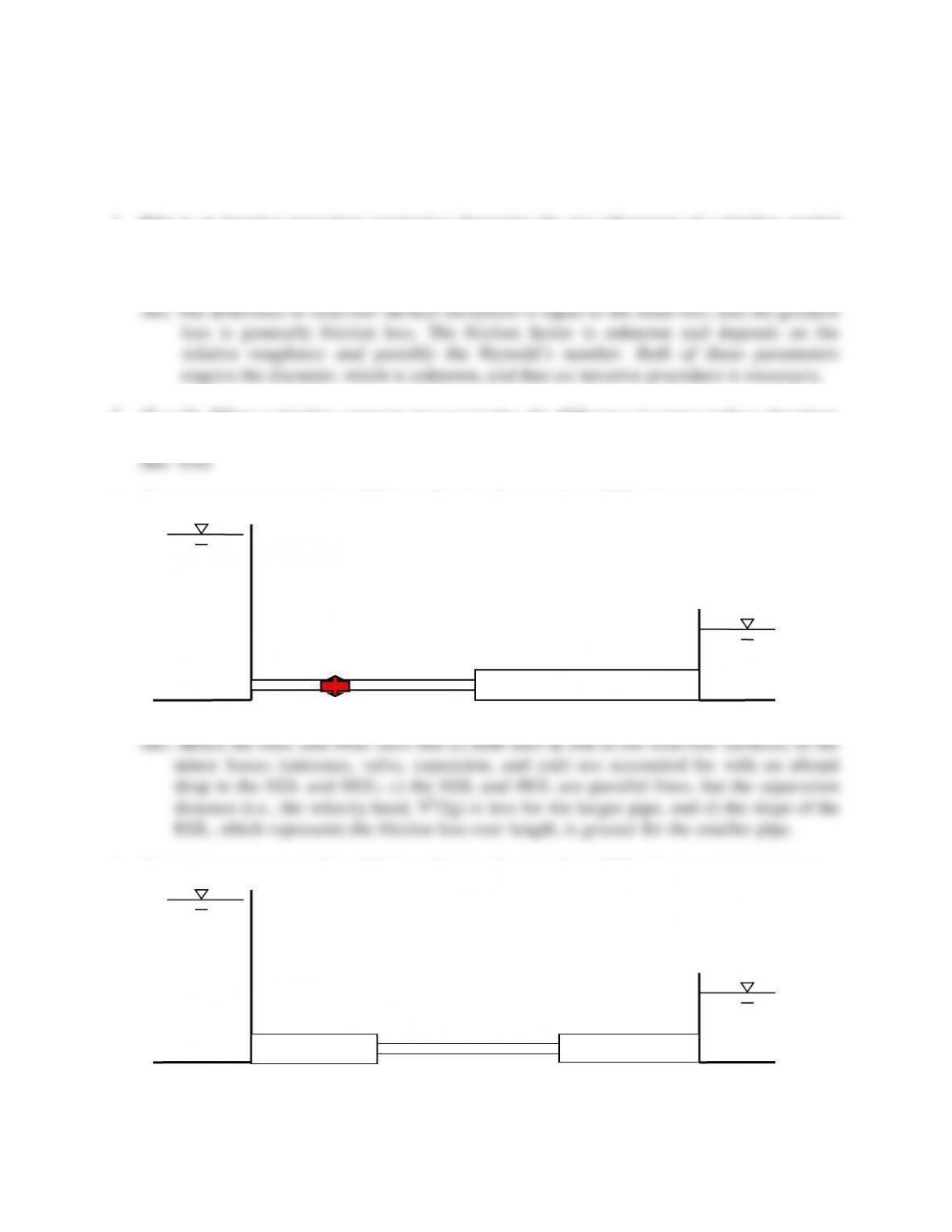27
TEST QUESTIONS & PROBLEMS - CHAPTER #4
1. Why is an iterative procedure required to determine the size (diameter) of a pipeline needed
to pass a given flow from one reservoir to another if you are provided with the reservoir
water surface elevations, pipe length, and the pipe material (assuming the Darcy-Weisbach
equation is used for friction loss)?
2. (T or F) When a pipeline connects two reservoirs, the difference in water surface elevations
is equal to the total head loss (friction and all minor losses).
3. Sketch the energy grade line (EGL) and hydraulic grade line (HGL) for the pipeline below.
4. Sketch the energy grade line (EGL) and hydraulic grade line (HGL) for the pipeline below.
Valve
ve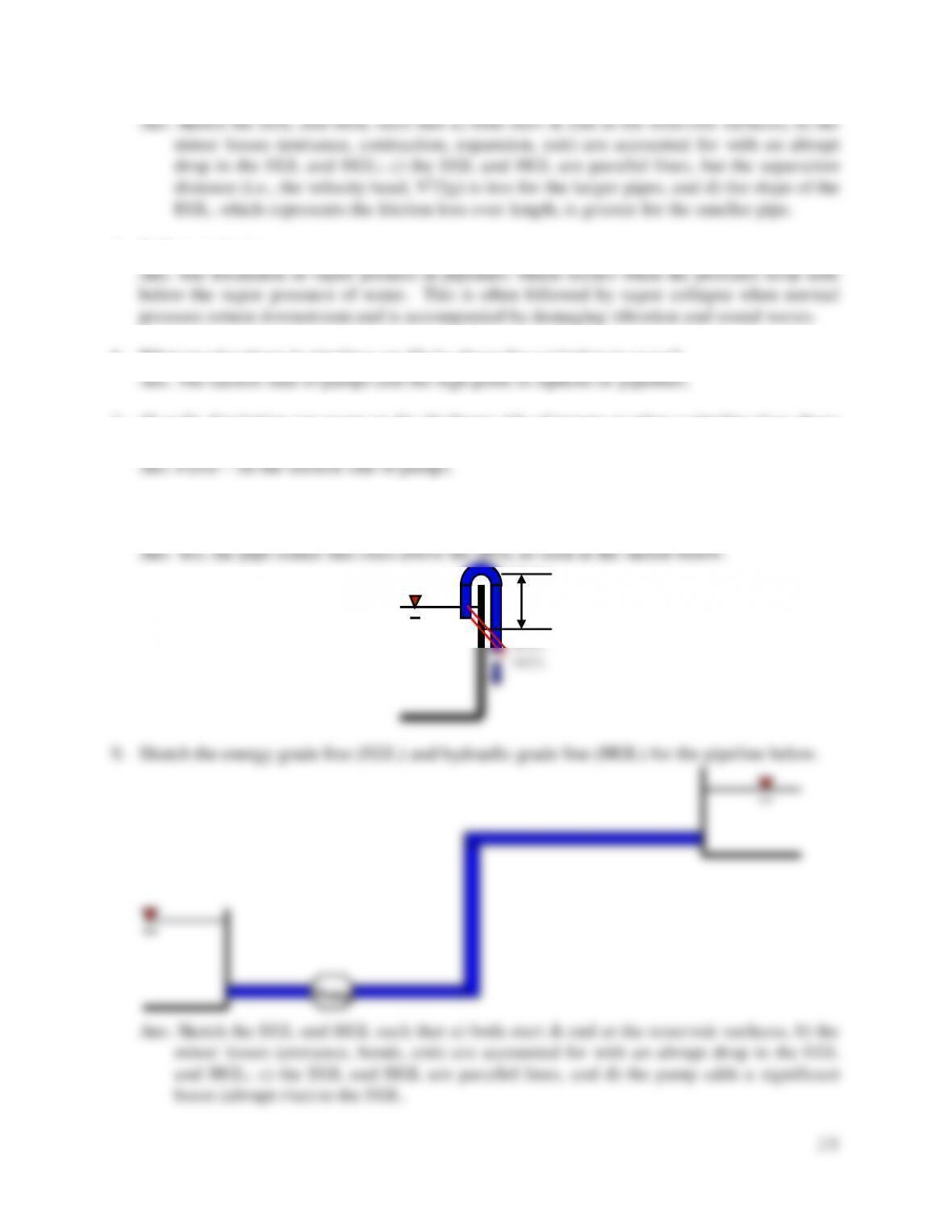28
5. Define cavitation.
6. What two locations in pipelines are likely places for cavitation to occur?
7. (T or F) Cavitation can occur on the discharge side of pumps or when a pipeline rises above
8. Do all siphons encounter negative pressure at their summit points? Prove your answer by
using an energy grade line (EGL) and hydraulic grade line (HGL) sketch.
9. Sketch the energy grade line (EGL) and hydraulic grade line (HGL) for the pipeline below.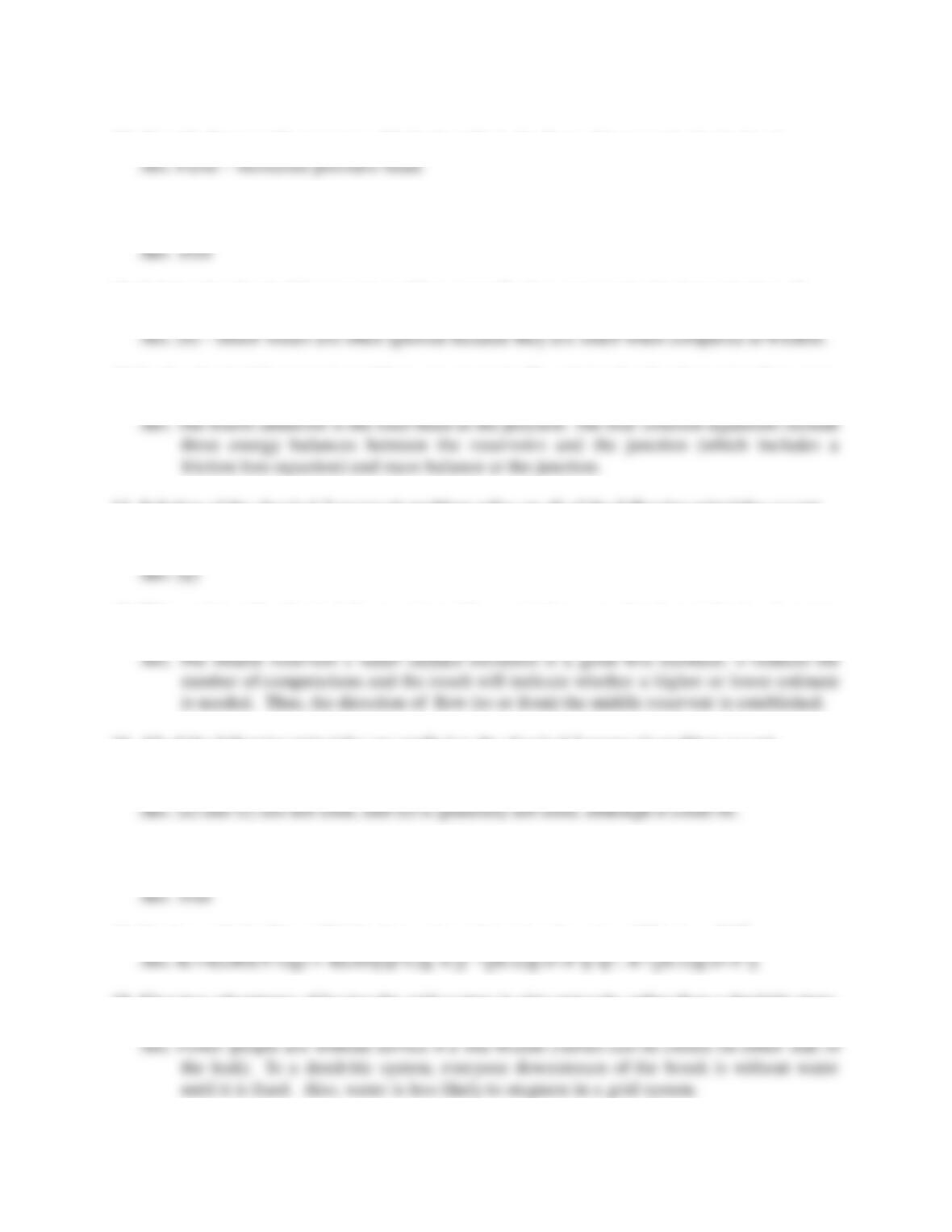10. (T or F) Pumps add energy to a fluid primarily in the form of increased velocity head.
11. (T or F) Negative gage pressure in a pipeline occurs whenever the pipe rises above the
12. Solving the classical 3-reservoir problem generally does not require the determination of
a) friction losses b) minor losses c) mass balance d) energy balance
13. In the classical 3-reservoir problem, we are typically solving for the three pipe flow rates.
What other unknown enters the analysis and what four equations are used in the solution?
14. Solution of the classical 3-reservoir problem relies on all of the following principles except
a) conservation of momentum b) conservation of energy
c) conservation of mass d) friction loss equation (e.g., Darcy-Weisbach)
15. When solving the classical 3-reservoir problem, a trial energy elevation at the junction may
be needed. What is a good first estimate and why?
16. All of the following principles are applied to the classical 3-reservoir problem except
b) conservation of momentum b) conservation of energy c) Bernoulli principle
d) conservation of mass e) friction losses e) minor losses
17. (T or F) Two major assumptions used in the solution of the classical 3-reservoir problem
include negligible minor losses in the pipes and a negligible velocity head at the junction.
18. Starting with the Darcy-Weisbach equation, determine the value of K in hf = KQ2.
19. Give two advantages of having the grid system in pipe networks rather than a dendritic (tree-
like) system in distributing water to urban customers.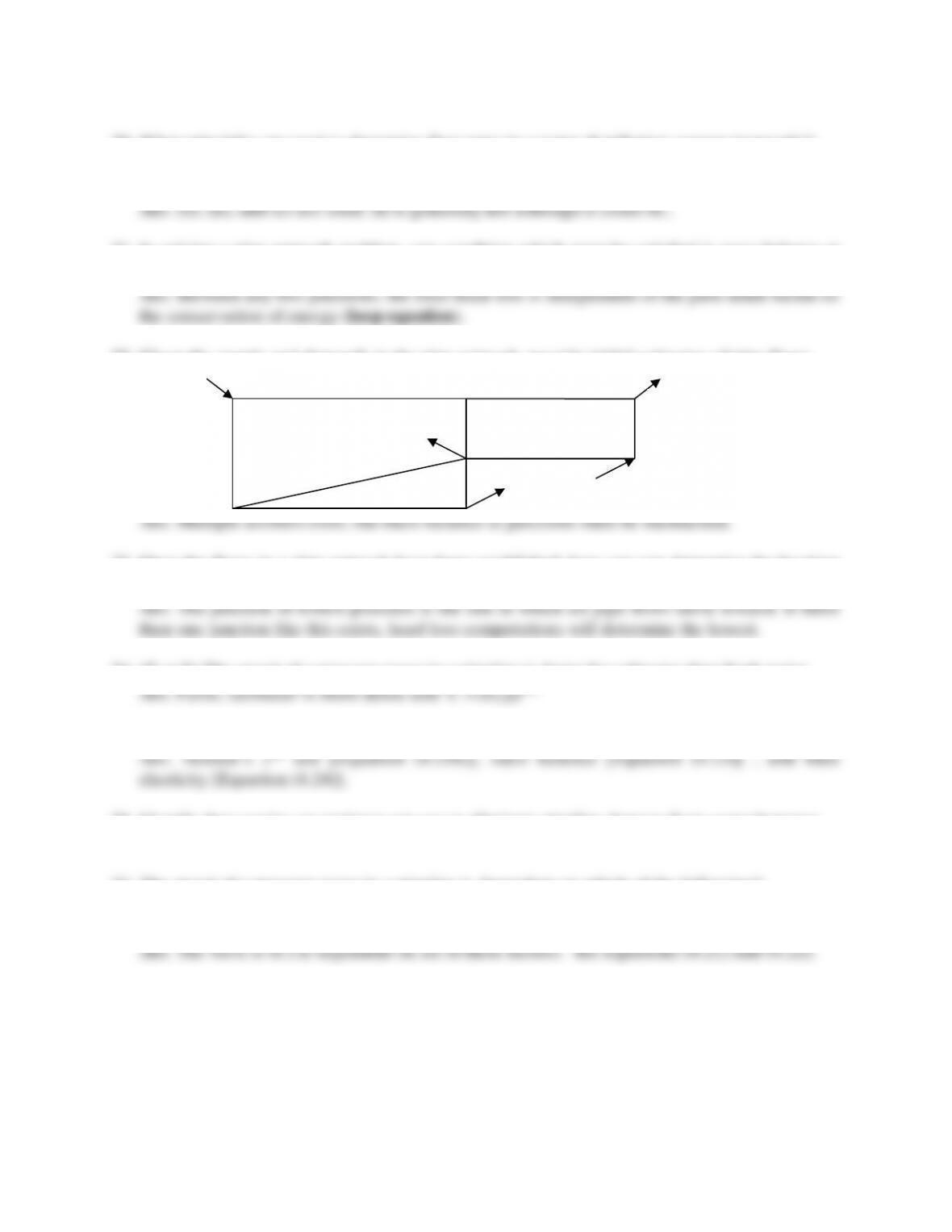30
20. What principles are used to determine flow rates in a water distribution system (network)?
c) conservation of momentum b) conservation of energy c) Bernoulli principle
d) conservation of mass e) friction losses e) minor losses
21. In solving a pipe network problem, one condition which must be satisfied is mass balance at
each junction (∑Q = 0). What other condition must be satisfied?
22. Given the supply and demands in the pipe network, provide initial estimates of pipe flows.
23. Once the flows in a pipe network have been established, how can you determine the location
of lowest pressure in the network if all junction elevations are the same?
24. (T or F) The speed of a pressure wave in a pipeline is faster for saltwater than fresh water.
25. Identify two principles used in the derivation of the water hammer equations for pipelines.
26. Identify three tactics an engineer can use to eliminate pipeline damage from water hammer.
Ans. Increase pipe wall thickness, slow valve closure, diverter, surge tank, or standpipe.
27. The speed of a pressure wave in a pipeline is dependent on which of the following?
a) fluid density b) pipe wall thickness c) pipe diameter
d) modulus of elasticity of the fluid e) pipeline anchoring method
150 cfs
100 cfs
100 cfs
100 cfs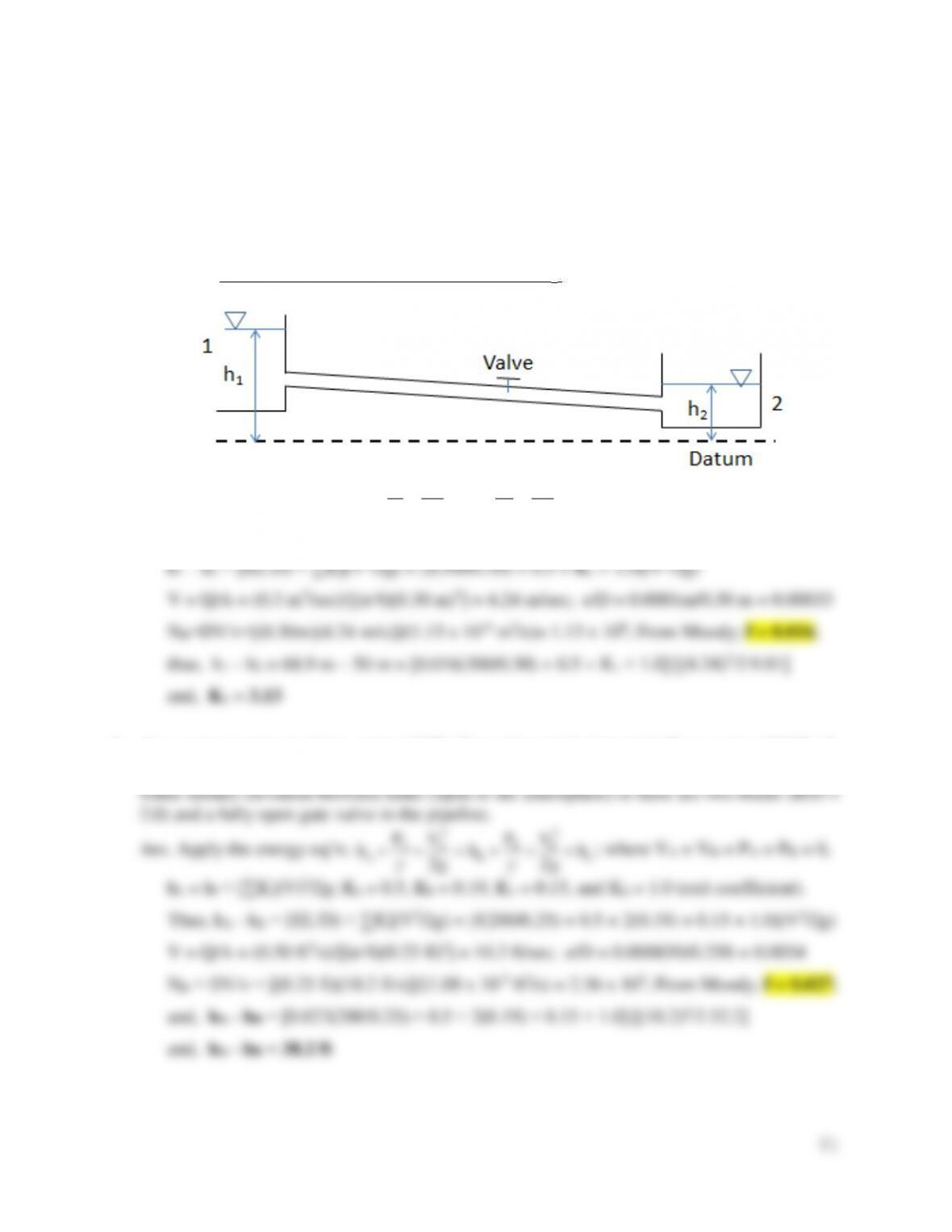31
Problems
1. In the pipe system shown below the pipe has a diameter of 30 cm, and it is 300 m long. The
equivalent roughness height of the pipe, e, is 0.1 mm. The kinematic viscosity is = 1.13 ×
10-6 m2/s. The minor head loss coefficients are: Ke = 0.5 for entrance losses, and Kd = 1.0 for
exit losses. The water surface elevations are h1= 68.9 m, and h2= 50 m. The discharge is Q =
0.30 m3/s. Determine the valve head loss coefficient, Kv.
Ans. Apply the energy eq’n;
L
h
g
VP
h
g
VP
h 22
2
22
2
2
11
1
; where V1 = V2 = P1 = P2 = 0,
hL = hf + [∑K](V)2/2g; Ke = 0.5, Kv = ?, Kd = 1.0 (exit coefficient). Thus,
2. At a water treatment plant, water (68˚F) flows from tank A to tank B at a rate of 0.50 cfs
through a 3-in.-diameter, cast-iron pipe that is 200 feet long. Determine the difference in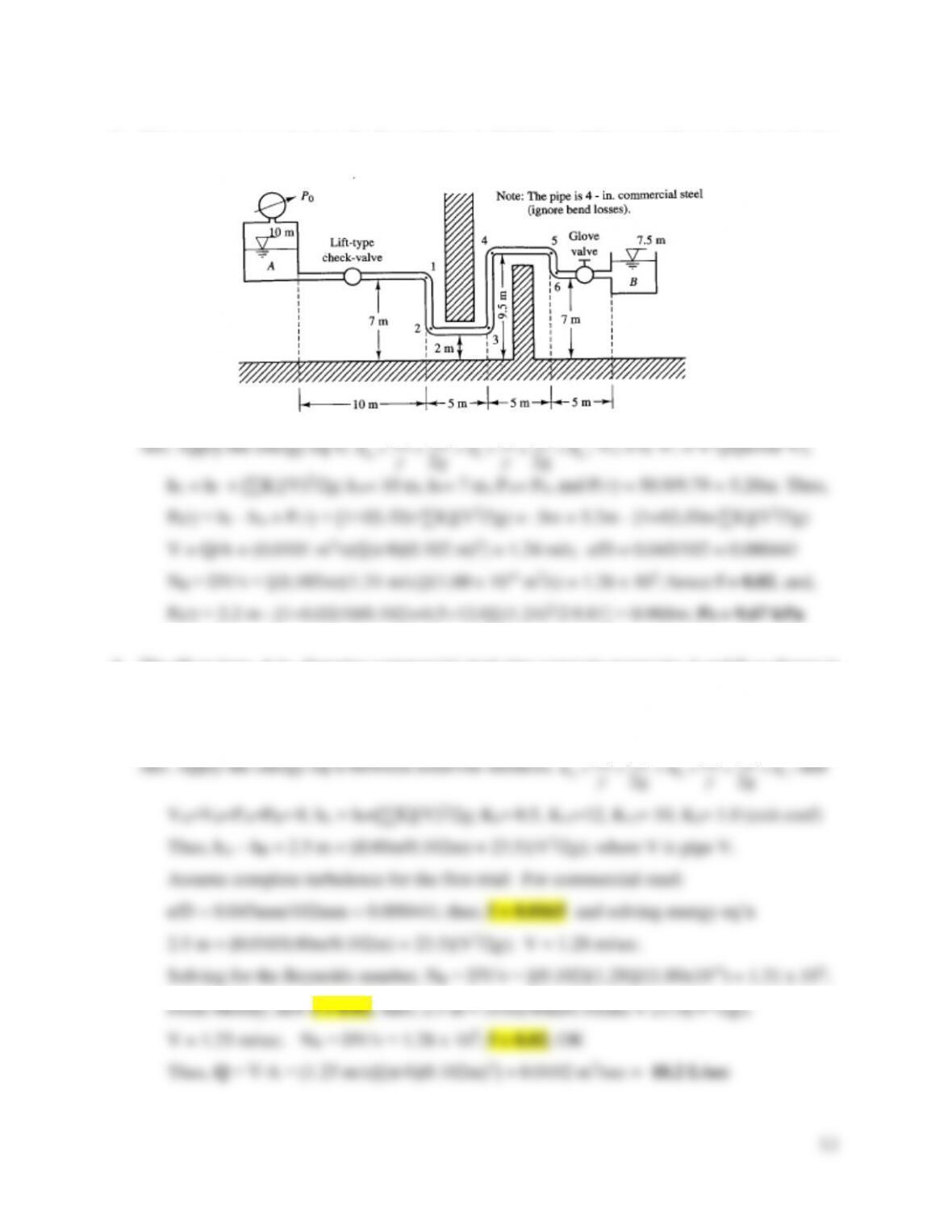3. If the pressure at point 1 in the figure below is 50.9 kPa and the water flow in the 4-inch pipe
(0.102 m) is 10.1 litres/sec (20°C),, determine the pressure P0 at reservoir A.
4. The 40-m-long, 4-in. diameter commercial steel pipe connects reservoirs A and B as shown in
the Figure from Problem #3 above. The water temperature is 20°C. Determine the flow rate
(liters/sec) if reservoir A is subjected to atmospheric pressure, the entrance is square-edged,
and bend losses are negligible.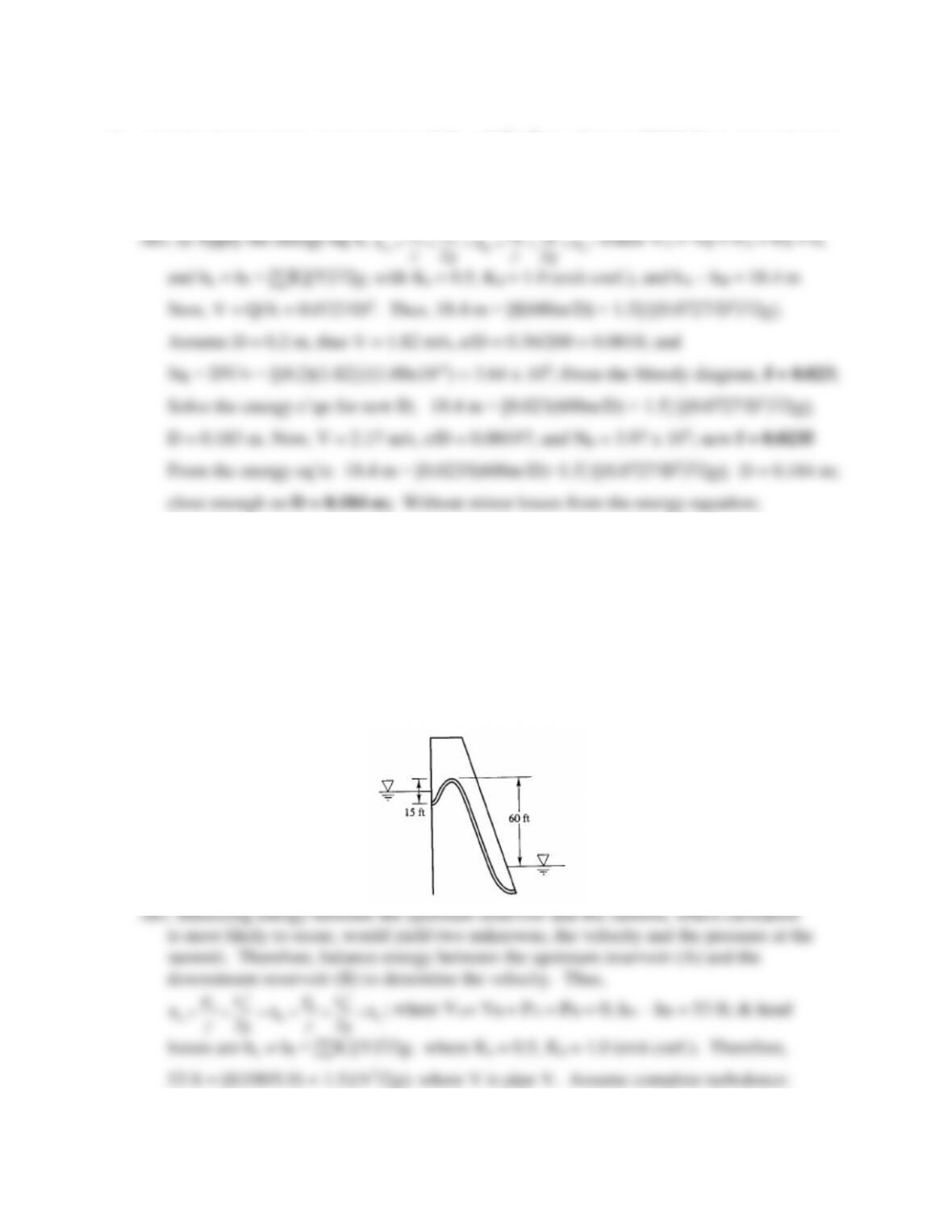5. An irrigation company must transport 5.71 x 10-2 m3/sec of water (β0˚C) from reservoir A to
reservoir B. The reservoirs are 600 m apart with an elevation difference of 18.4 m. Determine
the required pipe diameter if the roughness (e) of the pipe material is 0.36 mm. (Include
minor losses.) Would the solution differ if minor losses were neglected?
18.4 m = [0.0βγ5(600m/D)]∙[(0.07β7/D2)2/2g]; D = 0.183 m; essentially the same.
Note: The solution process is easier without minor losses; it is often a good idea to solve
this way first for an initial trial size and then solve again with minor losses included.
6. The siphon spillway shown below is 100 feet long with a 1.0-ft-diameter and conveys water
to a downstream reservoir. The friction losses in the rough concrete siphon are distributed
evenly throughout its length. If the siphon’s summit (high point) is 7 feet above the surface
of the upper reservoir and 18 feet (in pipe length) from the entrance, is cavitation (i.e., a
pressure less than two thirds of atmospheric pressure or -9.8 lb/in2) a concern?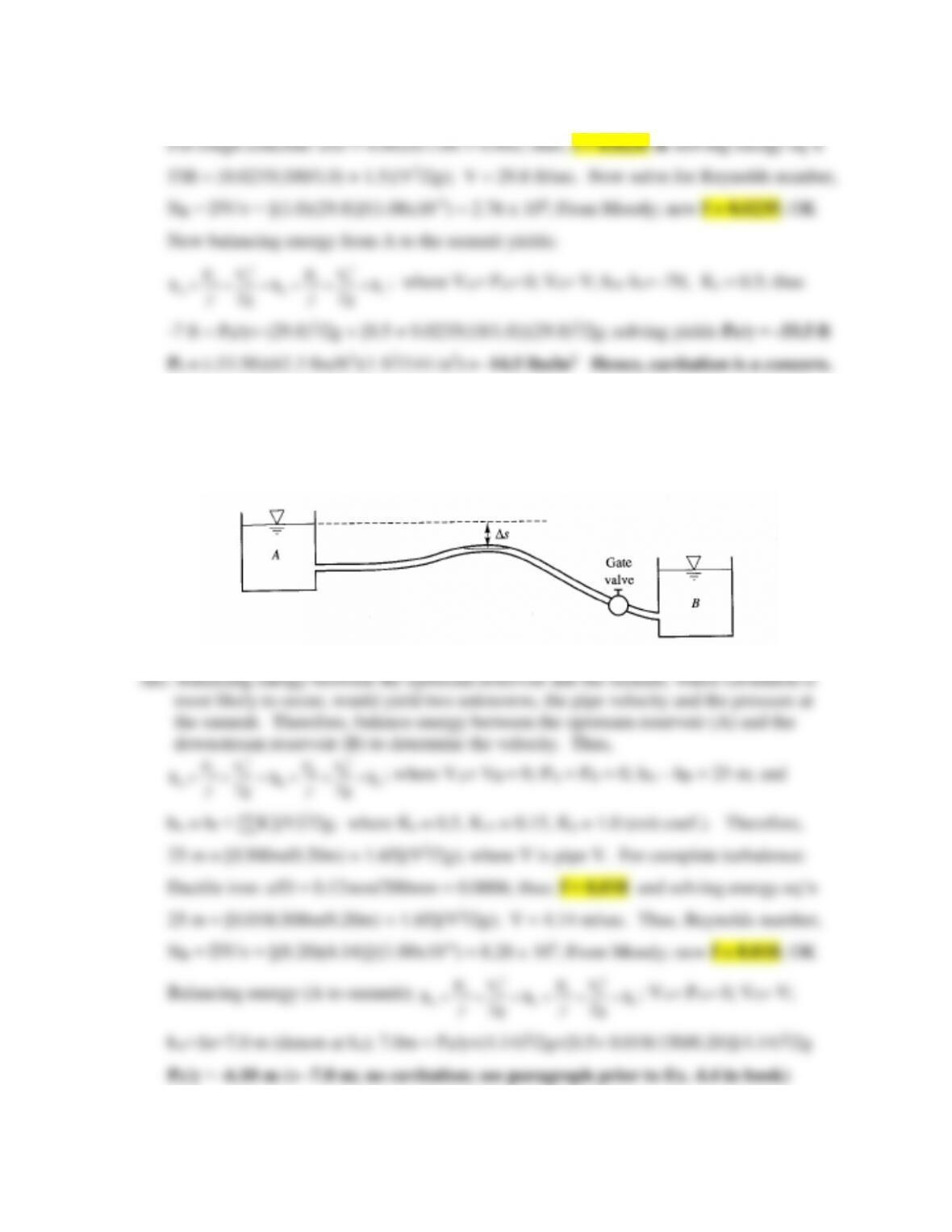34
7. Water flows in a new 20-cm, 300-m-long ductile iron pipe between reservoirs A and B, as
shown in the figure below. The pipe is elevated at S, which is 150 m downstream from
reservoir A. The water surface in reservoir B is 25 m below the water surface in reservoir A.
If Δs = 7.0 m, is cavitation a concern?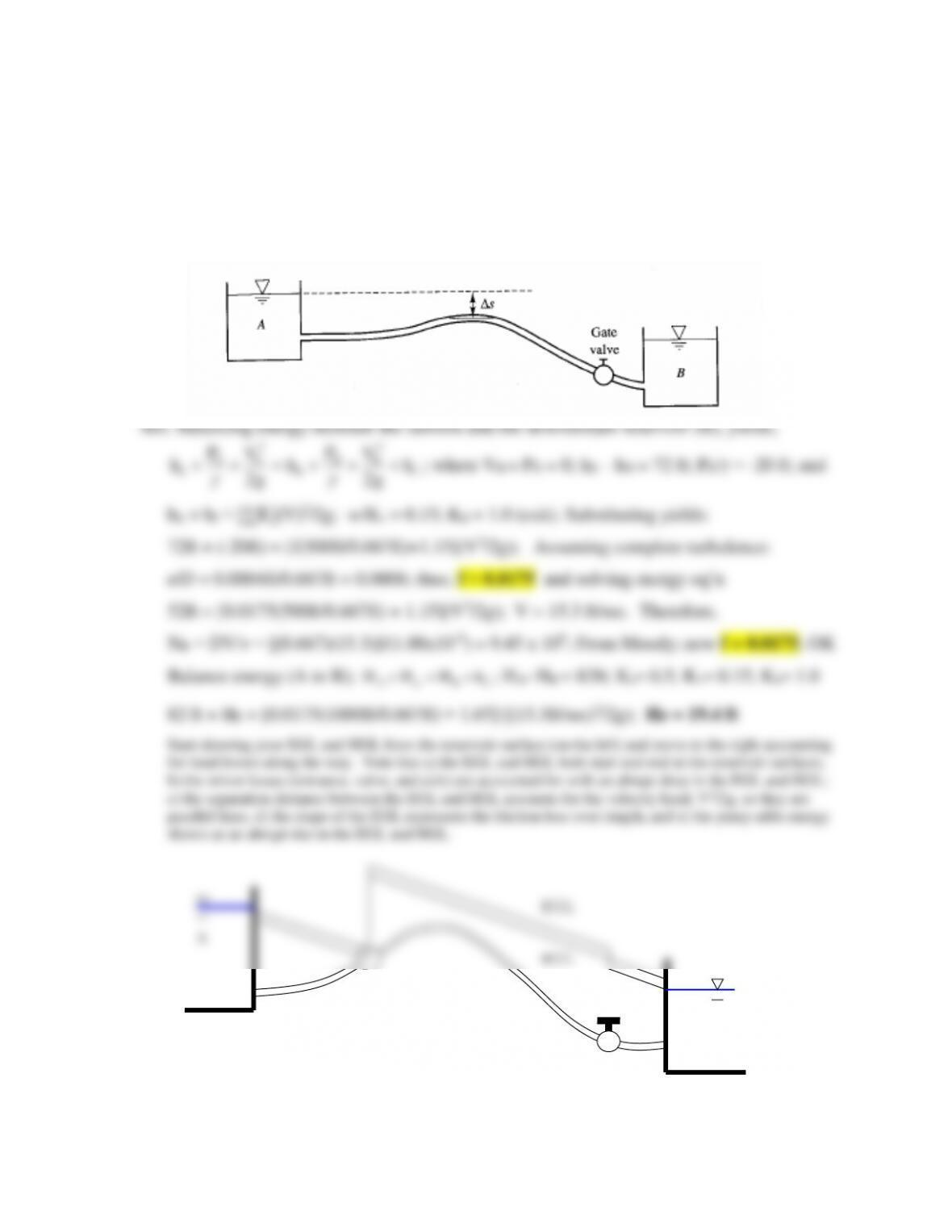35
8. Water flows in a new 8-in.-diameter, 1000-ft-long ductile iron pipe between reservoirs A and
B, as shown in the figure below. The pipe is elevated at S (location of the summit) which is
500 ft downstream from reservoir A. The water surface difference between the two reservoirs
is 82 ft. Determine the head that should be provided by a booster pump 330 feet downstream
from reservoir A if Δs is 10 feet and the pressure head at that location (summit) must be kept
above -20 feet due to cavitation concerns. Sketch the EGL and the HGL for the pipeline.

## Trusted by Thousands ofStudents

Here are what students say about us.